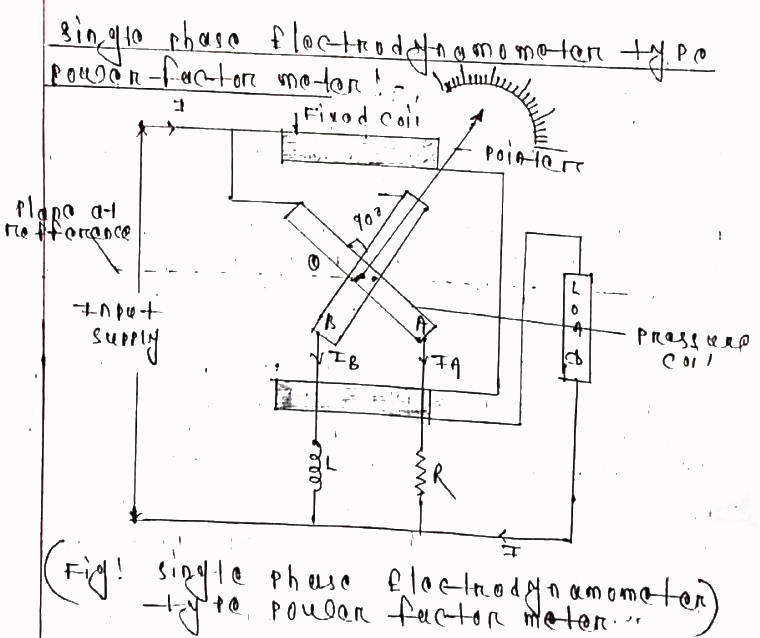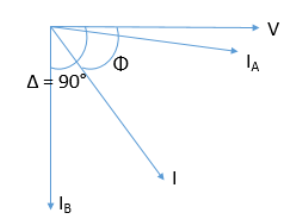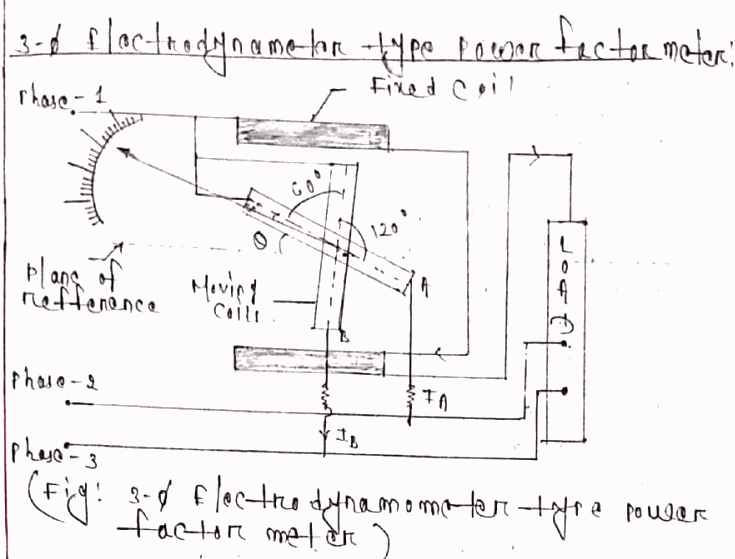### Tachometer

Definition: The power factor meter measures the power factor of a transmission system. The power factor is the cosine of the angle between the voltage and current. The power factor meter determines the types of load using on the line, and it also calculates the losses occur on it.

Construction:• The construction of single phase electrodynamometer Power Factor Meter is shown in figure It consists of a fixed coil which acts as the current coil.This coil is split up into two parts and carriers the current of the circuit under test.Therefore,the magnetic field produced by this coil is proportional to the main current.

• The single phase electrodynamometer type power factor consists of fixed coil and pressure coil.

• the fixed coil and splits into two parts and carries the currents of the circuit which is supplied to the load.The pressure coil also consists of two coils that is coil A and coil B.These two pressure coil pivoted on spindle which constitute the moving system.Pressurencoil ‘n’ is connected in series with inductance ‘L’.The current proportional to the voltage drop across the load flows through the pressure  coil.The value of R & Lare so adjusted that

• The angle between the plane of the coil is made equal to 90° The current Ib legs behind the voltage by 90° Current Ia is in phase with Voltage

• Working/Operation:In this case to deflecting Torque produced which acts on coil a and coil b the coils are so designed that the torque acting on it are equal and opposite in direction so the pointer takes a position where the two torquess are equal .

• Deflecting torque acting on coil A is:

Where,  = Angular Deflection from The Plan of Reference

= Maximun Value of Mutual Inductance Between the Two Coil

• Deflecting torque acting on coil B is:

• Therefore the deflection of the Instrument is the measure of phase angleOf the circuit The scale of the instrument can be calibrated Indirectly in the terms of power factor

• The phasor diagram is also shown For the circuit such that the cu

• rrent In the coil a is approximately At an angle of 90° To the current in the coil B## Three Phase Electro Dynamo type Power Factor MeterConstruction:
The above figure shows the construction and connection of a 3 phase electrodynamometer type power factor the two moving coils of the meter are shown and so placed that the angle between their place is is 120 degrees this two calls are connected across 2 different phases of the supply circuit each of these two coils are has series resistance through which it is connected to the phase angle the voltage applied across coil a is V12 and the current flowing in it is I a the voltage applied across coil B isV13 and the current flowing through it is Ib  these two moving coils are placed between segments of fixed coils

Working Principle:

• The basic working principle of power factor meter is similar to that of dynamometer type wattmeter that is when the field produced by a moving system tries to come in line with the field produced by the fixed coil deflecting torque is exerted on the moving system which deflects the pointer attached to it. Power factor meter is an indicating instrument and no controlling torque is provided in this instrument the currents are being led into the moving coil A and B by fine ligaments where exert no control.

• When the instrument is connected to the load circuit current flows through the fixed coil and moving coils A and B, flux is set by the fixed coil and moving coils.

• By the alignment of two fields, torque is developed that is the resultant field produced by the moving coil tries to come in line with the field produced by the fixed coil and the torque is developed still both of them come in line with each other there are three extreme conditions in which these instruments is connected in the circuit.

1. When power factor of the circuit is unity.

2. When power factor of the circuit is zero lagging

3. When power factor of the circuit is zero leading

Condition 1: when the power factor of the circuit is unity:

• In this case current is in phase with the circuit voltage the current flowing through potential coil A is in phase with the voltage which is also in phase with the current flowing through current coil FF at the same time the current flowing through the potential coil B lags behind the voltage as well as the current flowing through current coil FF be 90°.Thus pressure coil a will experience the turning moment so its plane will come in position a parallel to the plane of the current coil FF the torque acting on the pressure coil B is zero does the pointer indicates Unity power factor on the scale.

Condition 2: when the power factor of the circuit is zero lagging:

• In this case current lags behind the circuit voltage by 90° therefore the current flowing through pressure coil B will be in phase with the current in the circuit coil FF both being

• Lagging behind the circuit voltage by 90° .The current flowing through pressure coil A will lead the current in the current coil FF by 90° .Thus a turning moment acts on the pressure will be B and bring its plane parallel to the plane of current coil FF and the pointer indicates zero power factor lagging

Condition 3: Power factor of the circuit is zero leading:

• In this case current leads the circuit voltage by 90° therefore the current flowing through the pressure coil A lags the current  in the current coil FF by 90° and current flowing through the pressure will B lags behind the current in the current coil FF by  180 degrees. Thus the field produced by the moving system is just reversed to that in the case( 2).Thus an opposite turning moment acts on the pressure coil B and brings its plane parallel to the plane of the current coil asFF and the pointer indicates zero power factor leading.

## Power Factor Meter Types

The power factor meter is of two types they are

• Electrodynamometer:

1. Single Phase Electrodynamometer

2. Three Phase Electrodynamometer

• ​​​​​​​Moving Iron Type Meter:

1. Rotating Iiron magnetic field

2. Number of alternating field